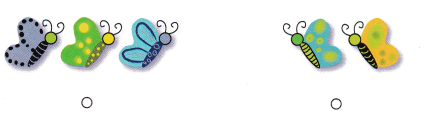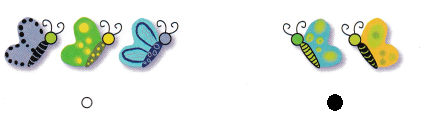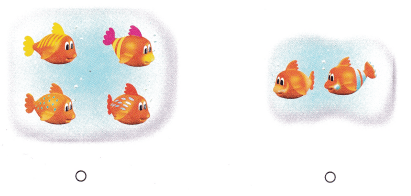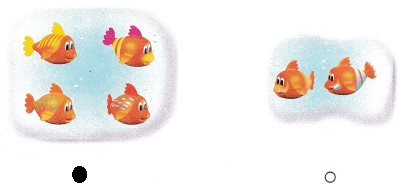# Texas Go Math Kindergarten Lesson 1.4 Answer Key Understand Numbers 1 Through 4

Refer to our Texas Go Math Kindergarten Answer Key Pdf to score good marks in the exams. Test yourself by practicing the problems from Texas Go Math Kindergarten Lesson 1.4 Answer Key Understand Numbers 1 Through 4.

## Texas Go Math Kindergarten Lesson 1.4 Answer Key Understand Numbers 1 Through 4

Unlock the Problem

DIRECTIONS: Use counters to model this problem. There are two ducks in the pond. One more duck comes n. Count the ducks in the pond now. Write the number. Explain how you used counters to model that number.Explanation:
There are 2 ducks in the pond
one more duck joined
so, it become 3. Written the numbers with the help of counters

Try Another Problem

DIRECTIONS: Use counters, or select an iTool, to model the baskets. 1. Two children each have one basket for a picnic. Draw the counters. How many baskets are there? Write the number. 2. Four children each bring one apple for a picnic. Use counters to show the apples. Draw the counters. How many apples are there? Write the number.

Question 1.Explanation:
Two children each have one basket for a picnic.
Drawn the counters to show number 2.

Question 2.Explanation:
Four children each bring one apple for a picnic.
so, there are 4 apples
Used counters to show the number of apples with the help of counters

Show and Show

DIRECTIONS: Use counters, or select an iTool, to model and draw the problem. 3. Gus has 3 cookies. He eats1 cookie and gives 1 cookie to his friend. How many cookies does Gus have now? Write the number. 4. Ella has 4- carrot sticks. She gives 1 away. How many carrot sticks does Ella have now? Write the number.

Question 3.Explanation:
3 – 2 = 1

Question 4.Explanation:
Ella has 4- carrot sticks.
She gives 1 away.
4 – 1 = 3
Ella have 3 carrot sticks

HOME ACTIVITY • Give your child 2 to 4 small items such as beans. Hove your child count the items and tell you how many.
Answer: There are 4 peas when we have given the peas to count

DIRECTIONS Choose the correct answer. 5. Han has 3 books He gives 1 away. How many books does Kan have now? 6. Which set has 4 blocks? 7. Which set hos 3 pencils?

Question 5.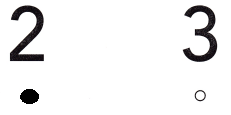Explanation:
Han has 3 books He gives 1 away.
3 – 1 = 2
Kan have 2 books

Question 6.Explanation:
Second set has 4 blocks
bubbled the correct one

Question 7.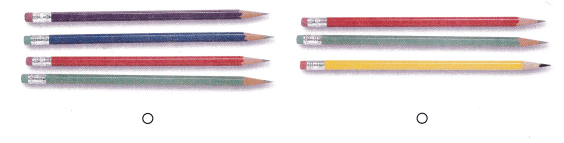Explanation:
Bubbled the second set it has 3 pencils

### Texas Go Math Kindergarten Lesson 1.4 Homework and Practice Answer Key

DIRECTIONS: Draw counters, or select on iTool. to model each problem. 1. There are three birds in a tree. One bird flies away. How many birds are in the tree now? Write the number. 2. John has 2 balloons, His friend gives him 2 more balloons How many balloons does John have now? Write the number.

Question 1.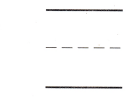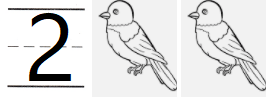Explanation:
There are three birds in a tree.
One bird flies away.
3 – 1 = 2
there are 2 birds.

Question 2.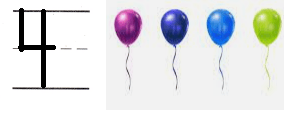Explanation:
John has 2 balloons,
His friend gives him 2 more balloons
2 + 2 = 4
Jhon has 4 balloons

DIRECTIONS: Choose the correct answer. 3. Parker has 4 hats. He gives one away. How many hats does Parker have now? 4. Which set has 2 butterflies? 5. Which set has 4 fish?

Lesson Check

Question 3.Explanation:
Parker has 4 hats.
He gives one away.
4 – 1 = 3
Parker has 3 hats now

Question 4.